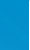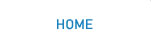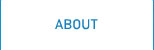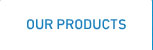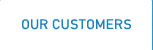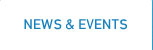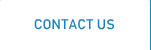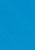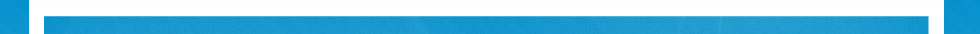# independent third-party test results

#### Project No. 148 Test Report for the ARID Technologies Vapor Recovery Unit installed in LANTANA, FL, continued

Prepared for: Arid Technologies, Inc.
Prepared By: Matus Technical Services, Inc.

#### 5.0 Calculations

The outlet hydrocarbon (HC) mass is calculated from the measured outlet volume corrected to standard conditions, the outlet HC concentration as propane and the density of propane. Standard conditions are 68 degrees F and 29.92 inches Hg.

The outlet volume during any one minute test interval is corrected to standard conditions using the formula: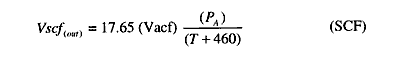Where:
17.65 = Standard Temperature / Standard Pressure
Vacf = Recorded meter volume in cubic feet
PA = Absolute pressure (barometric plus turbine meter) in inches of mercury
T = Temperature in volume meter in F

The mass of hydrocarbons emitted from the outlet during any one minute test period is calculated from: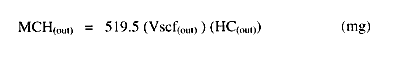Where:
HC(out) = Hydrocarbon concentration at the outlet in %C3
Note: The density of propane is 51,950 mg/ft3

For recovery efficiency of the PERMEATOR®, the feed volume is calculated from the outlet volume, outlet hydrocarbon concentration, permeate hydrocarbon concentration and inlet hydrocarbon concentration.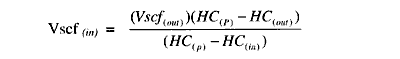Where:
HC(in) = Hydrocarbon concentration at the inlet in %C3 HC(P) = Hydrocarbon concentration at the permeate in %C3

The inlet mass during any one minute test interval is: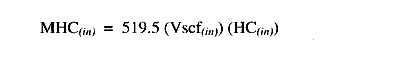The hydrocarbon removal efficiency is calculated from the inlet and outlet mass. Efficiency can be calculated on an hourly basis using the total mass in and out for the hour or overall for the entire test period using the overall mass in and out.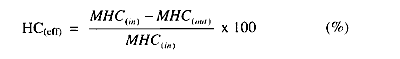The gallonage weighted average emission is calculated from the total amount of hydrocarbons emitted divided by the total amount of gasoline dispensed.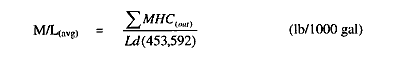Where:
Ld = Gasoline loaded in gallons.

For recovery efficiency of the PERMEATOR®, the feed volume is calculated from the outlet volume, outlet hydrocarbon concentration, permeate hydrocarbon concentration and inlet hydrocarbon concentration.Where:
HC(in) = Hydrocarbon concentration at the inlet in %C3 HC(P) = Hydrocarbon concentration at the permeate in %C3

Go to Matus Report page:    1234